# Dereverberate Speech Using Deep Learning Networks

This example shows how to train a U-Net fully convolutional network (FCN)  to dereverberate a speech signals.

### Introduction

Reverberation occurs when a speech signal is reflected off objects in space, causing multiple reflections to build up and eventually leads to degradation of speech quality. Dereverberation is the process of reducing the reverberation effects in a speech signal.

### Dereverberate Speech Signal Using Pretrained Network

Before going into the training process in detail, use a pretrained network to dereverberate a speech signal.

Download the pretrained network. This network was trained on 56-speaker versions of the training datasets. The example walks through training on the 28-speaker version.

```downloadFolder = matlab.internal.examples.downloadSupportFile("audio","dereverbnet.zip"); dataFolder = tempdir; unzip(downloadFolder,dataFolder) netFolder = fullfile(dataFolder,"derevernet"); load(fullfile(netFolder,"dereverbNet.mat"));```

Listen to a clean speech signal sampled at 16 kHz.

```[cleanAudio,fs] = audioread("clean_speech_signal.wav"); sound(cleanAudio,fs)```

An acoustic path can be modelled using a room impulse response. You can model reverberation by convolving an anechoic signal with a room impulse response.

Load and plot a room impulse response.

```[rirAudio,fsR] = audioread("room_impulse_response.wav"); tAxis = (1/fsR)*(0:numel(rirAudio)-1); figure plot(tAxis,rirAudio) xlabel("Time (s)") ylabel("Amplitude") grid on```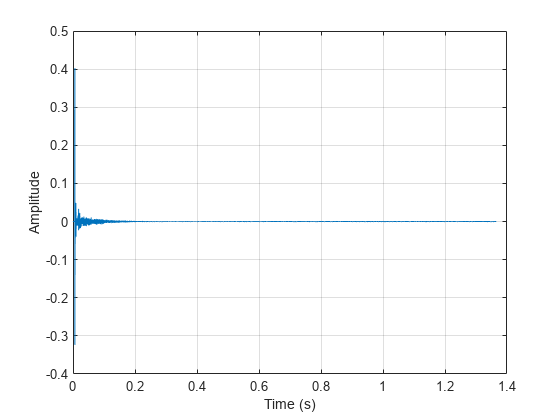Convolve the clean speech with the room impulse response to obtain reverberated speech. Align the lengths and amplitudes of the reverberated and clean speech signals.

```revAudio = conv(cleanAudio,rirAudio); revAudio = revAudio(1:numel(cleanAudio)); revAudio = revAudio.*(max(abs(cleanAudio))/max(abs(revAudio)));```

Listen to the reverberated speech signal.

`sound(revAudio,fs)`

The input to the pretrained network is the log-magnitude short-time Fourier transform (STFT) of the reverberant audio. The network predicts the log-magnitude STFT of the dereverberated input. To estimate the original time-domain audio signal, you perform an inverse STFT and assume the phase of the reverberant audio.

Use the following parameters to compute the STFT.

```params.WindowdowLength = 512; params.Window = hamming(params.WindowdowLength,"periodic"); params.OverlapLength = round(0.75*params.WindowdowLength); params.FFTLength = params.WindowdowLength;```

Use `stft` to compute the one-sided log-magnitude STFT. Use single precision when computing features to better utilize memory usage and to speed up the training. Even though the one-sided STFT yields 257 frequency bins, consider only 256 bins and ignore the highest frequency bin.

```revAudio = single(revAudio); audioSTFT = stft(revAudio,Window=params.Window,OverlapLength=params.OverlapLength, ... FFTLength=params.FFTLength,FrequencyRange="onesided"); Eps = realmin("single"); reverbFeats = log(abs(audioSTFT(1:end-1,:)) + Eps);```

Extract the phase of the STFT.

`phaseOriginal = angle(audioSTFT(1:end-1,:));`

Each input will have dimensions 256-by-256 (frequency bins by time steps). Split the log-magnitude STFT into segments of 256 time-steps.

```params.NumSegments = 256; params.NumFeatures = 256; totalFrames = size(reverbFeats,2); chunks = ceil(totalFrames/params.NumSegments); reverbSTFTSegments = mat2cell(reverbFeats,params.NumFeatures, ... [params.NumSegments*ones(1,chunks - 1),(totalFrames - (chunks-1)*params.NumSegments)]); reverbSTFTSegments{chunks} = reverbFeats(:,end-params.NumSegments + 1:end);```

Scale the segmented features to the range [-1,1]. Retain the minimum and maximum values used to scale for reconstructing the dereverberated signal.

```minVals = num2cell(cellfun(@(x)min(x,[],"all"),reverbSTFTSegments)); maxVals = num2cell(cellfun(@(x)max(x,[],"all"),reverbSTFTSegments)); featNorm = cellfun(@(feat,minFeat,maxFeat)2.*(feat - minFeat)./(maxFeat - minFeat) - 1, ... reverbSTFTSegments,minVals,maxVals,UniformOutput=false);```

Reshape the features so that chunks are along the fourth dimension.

`featNorm = reshape(cell2mat(featNorm),params.NumFeatures,params.NumSegments,1,chunks);`

Predict the log-magnitude spectra of the reverberated speech signal using the pretrained network.

`predictedSTFT4D = predict(dereverbNet,featNorm);`

Reshape to 3-dimensions and scale the predicted STFTs to the original range using the saved minimum-maximum pairs.

```predictedSTFT = squeeze(mat2cell(predictedSTFT4D,params.NumFeatures,params.NumSegments,1,ones(1,chunks)))'; featDeNorm = cellfun(@(feat,minFeat,maxFeat) (feat + 1).*(maxFeat-minFeat)./2 + minFeat, ... predictedSTFT,minVals,maxVals,UniformOutput=false);```

Reverse the log-scaling.

`predictedSTFT = cellfun(@exp,featDeNorm,UniformOutput=false);`

Concatenate the predicted 256-by-256 magnitude STFT segments to obtain the magnitude spectrogram of original length.

```predictedSTFTAll = predictedSTFT(1:chunks - 1); predictedSTFTAll = cat(2,predictedSTFTAll{:}); predictedSTFTAll(:,totalFrames - params.NumSegments + 1:totalFrames) = predictedSTFT{chunks};```

Before taking the inverse STFT, append zeros to the predicted log-magnitude spectrum and the phase in lieu of the highest frequency bin which was excluded when preparing input features.

```nCount = size(predictedSTFTAll,3); predictedSTFTAll = cat(1,predictedSTFTAll,zeros(1,totalFrames,nCount)); phase = cat(1,phaseOriginal,zeros(1,totalFrames,nCount));```

Use the inverse STFT function to reconstruct the dereverberated time-domain speech signal using the predicted log-magnitude STFT and the phase of reverberant speech signal.

```oneSidedSTFT = predictedSTFTAll.*exp(1j*phase); dereverbedAudio = istft(oneSidedSTFT, ... Window=params.Window,OverlapLength=params.OverlapLength, ... FFTLength=params.FFTLength,ConjugateSymmetric=true, ... FrequencyRange="onesided"); dereverbedAudio = dereverbedAudio./max(abs([dereverbedAudio;revAudio])); dereverbedAudio = [dereverbedAudio;zeros(length(revAudio) - numel(dereverbedAudio), 1)];```

Listen to the dereverberated audio signal.

`sound(dereverbedAudio,fs)`

Plot the clean, reverberant, and dereverberated speech signals.

```t = (1/fs)*(0:numel(cleanAudio)-1); figure tiledlayout(3,1) nexttile plot(t,cleanAudio) xlabel("Time (s)") grid on subtitle("Clean Speech Signal") nexttile plot(t,revAudio) xlabel("Time (s)") grid on subtitle("Revereberated Speech Signal") nexttile plot(t,dereverbedAudio) xlabel("Time (s)") grid on subtitle("Derevereberated Speech Signal")```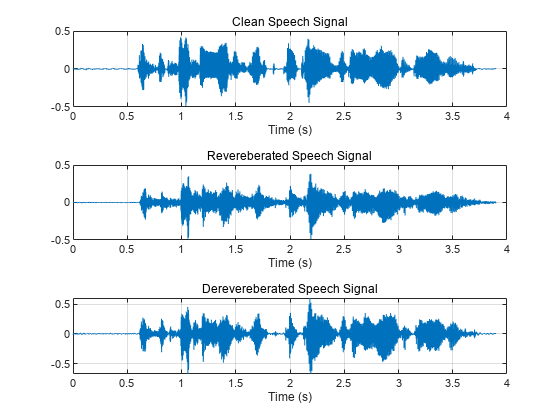Visualize the spectrograms of the clean, reverberant, and dereverberated speech signals.

```figure(Position=[100,100,800,800]) tiledlayout(3,1) nexttile spectrogram(cleanAudio,params.Window,params.OverlapLength,params.FFTLength,fs,"yaxis"); subtitle("Clean") nexttile spectrogram(revAudio,params.Window,params.OverlapLength,params.FFTLength,fs,"yaxis"); subtitle("Reverberated") nexttile spectrogram(dereverbedAudio,params.Window,params.OverlapLength,params.FFTLength,fs,"yaxis"); subtitle("Predicted (Dereverberated)")```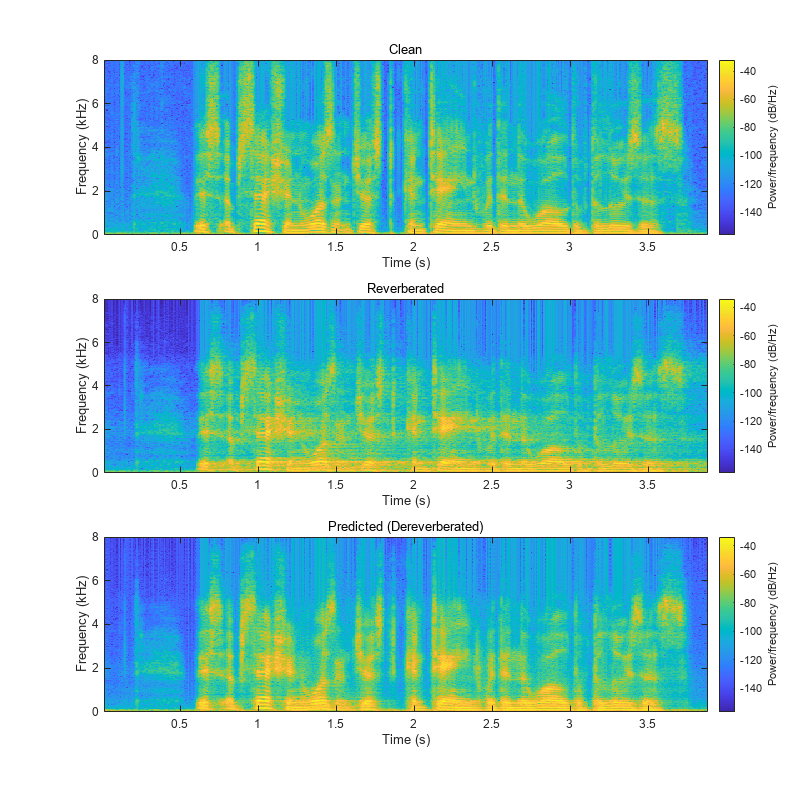This example uses the Reverberant Speech Database  and the corresponding Clean Speech Database  to train the network.

```url1 = "https://datashare.is.ed.ac.uk/bitstream/handle/10283/2791/clean_trainset_28spk_wav.zip"; url2 = "https://datashare.is.ed.ac.uk/bitstream/handle/10283/2791/clean_testset_wav.zip"; downloadFolder = tempdir; cleanDataFolder = fullfile(downloadFolder,"DS_10283_2791"); if ~datasetExists(cleanDataFolder) disp("Downloading data set (6 GB) ...") unzip(url1,cleanDataFolder) unzip(url2,cleanDataFolder) end```
```Downloading data set (6 GB) ... ```

```url3 = "https://datashare.is.ed.ac.uk/bitstream/handle/10283/2031/reverb_trainset_28spk_wav.zip"; url4 = "https://datashare.is.ed.ac.uk/bitstream/handle/10283/2031/reverb_testset_wav.zip"; downloadFolder = tempdir; reverbDataFolder = fullfile(downloadFolder,"DS_10283_2031"); if ~datasetExists(reverbDataFolder) disp("Downloading data set (6 GB) ...") unzip(url3,reverbDataFolder) unzip(url4,reverbDataFolder) end```
```Downloading data set (6 GB) ... ```

### Data Preprocessing and Feature Extraction

1. Synthetically generate reverberant data using the `reverberator` object

2. Split each speech signal into small segments of 2.072s duration

3. Discard segments which contain significant silent regions

4. Extract log-magnitude STFTs as predictor and target features

5. Scale and reshape features

First, create two `audioDatastore` objects that point to the clean and reverberant speech datasets.

```adsCleanTrain = audioDatastore(fullfile(cleanDataFolder,"clean_trainset_28spk_wav"),IncludeSubfolders=true); adsReverbTrain = audioDatastore(fullfile(reverbDataFolder,"reverb_trainset_28spk_wav"),IncludeSubfolders=true);```

#### Synthetic Reverberant Speech Data Generation

The amount of reverberation in the original data is relatively small. You will augment the reverberant speech data with significant reverberation effects using the `reverberator` object.

Create an `audioDatastore` that points to the clean speech dataset allocated for synthetic reverberant data generation.

```adsSyntheticCleanTrain = subset(adsCleanTrain,10e3+1:length(adsCleanTrain.Files)); adsCleanTrain = subset(adsCleanTrain,1:10e3); adsReverbTrain = subset(adsReverbTrain,1:10e3);```

Resample from 48 kHz to 16 kHz.

```adsSyntheticCleanTrain = transform(adsSyntheticCleanTrain,@(x)resample(x,16e3,48e3)); adsCleanTrain = transform(adsCleanTrain,@(x)resample(x,16e3,48e3)); adsReverbTrain = transform(adsReverbTrain,@(x)resample(x,16e3,48e3));```

Combine the two audio datastores, maintaining the correspondence between the clean and reverberant speech samples.

`adsCombinedTrain = combine(adsCleanTrain,adsReverbTrain);`

The applyReverb function creates a `reverberator` object, updates the pre delay, decay factor, and wet-dry mix parameters as specified, and then applies reverberation. Use `audioDataAugmenter` to create synthetically generated reverberant data.

```augmenter = audioDataAugmenter(AugmentationMode="independent",NumAugmentations=1,ApplyAddNoise=0, ... ApplyTimeStretch=0,ApplyPitchShift=0,ApplyVolumeControl=0,ApplyTimeShift=0); algorithmHandle = @(y,preDelay,decayFactor,wetDryMix,samplingRate) ... applyReverb(y,preDelay,decayFactor,wetDryMix,samplingRate); addAugmentationMethod(augmenter,"Reverb",algorithmHandle, ... AugmentationParameter={'PreDelay','DecayFactor','WetDryMix','SamplingRate'}, ... ParameterRange={[0.15,0.25],[0.2,0.5],[0.3,0.45],[16000,16000]}) augmenter.ReverbProbability = 1; disp(augmenter)```
``` audioDataAugmenter with properties: AugmentationMode: "independent" AugmentationParameterSource: 'random' NumAugmentations: 1 ApplyTimeStretch: 0 ApplyPitchShift: 0 ApplyVolumeControl: 0 ApplyAddNoise: 0 ApplyTimeShift: 0 ApplyReverb: 1 PreDelayRange: [0.1500 0.2500] DecayFactorRange: [0.2000 0.5000] WetDryMixRange: [0.3000 0.4500] SamplingRateRange: [16000 16000] ```

Create a new `audioDatastore` corresponding to synthetically generated reverberant data by calling `transform` to apply data augmentation.

`adsSyntheticReverbTrain = transform(adsSyntheticCleanTrain,@(y)deal(augment(augmenter,y,16e3).Audio{1}));`

Combine the two audio datastores.

`adsSyntheticCombinedTrain = combine(adsSyntheticCleanTrain,adsSyntheticReverbTrain);`

Next, based on the dimensions of the input features to the network, segment the audio into chunks of 2.072 s duration with an overlap of 50%.

Having too many silent segments can adversely affect the DNN model training. Remove the segments which are mostly silent (more than 50% of the duration) and exclude those from the model training. Do not completely remove silence because the model will not be robust to silent regions and slight reverberation effects could be identified as silence. `detectSpeech` can identify the start and end points of silent regions. After these two steps, the feature extraction process can be carried out as explained in the first section. helperFeatureExtract implements these steps.

Define the feature extraction parameters. By setting `speedupExample` to `true`, you choose a small subset of the datasets to perform the subsequent steps.

```speedupExample =true; params.fs = 16000; params.WindowdowLength = 512; params.Window = hamming(params.WindowdowLength,"periodic"); params.OverlapLength = round(0.75*params.WindowdowLength); params.FFTLength = params.WindowdowLength; samplesPerMs = params.fs/1000; params.samplesPerImage = (24+256*8)*samplesPerMs; params.shiftImage = params.samplesPerImage/2; params.NumSegments = 256; params.NumFeatures = 256```
```params = struct with fields: WindowdowLength: 512 Window: [512×1 double] OverlapLength: 384 FFTLength: 512 NumSegments: 256 NumFeatures: 256 fs: 16000 samplesPerImage: 33152 shiftImage: 16576 ```

To speed up processing, distribute the preprocessing and feature extraction task across multiple workers using `parfor`.

Determine the number of partitions for the dataset. If you do not have Parallel Computing Toolbox, use a single partition.

```if ~isempty(ver("parallel")) pool = gcp; numPar = numpartitions(adsCombinedTrain,pool); else numPar = 1; end```
```Starting parallel pool (parpool) using the 'local' profile ... Connected to the parallel pool (number of workers: 6). ```

For each partition, read from the datastore, preprocess the audio signal, and then extract the features.

```if speedupExample adsCombinedTrain = shuffle(adsCombinedTrain); adsCombinedTrain = subset(adsCombinedTrain,1:200); adsSyntheticCombinedTrain = shuffle(adsSyntheticCombinedTrain); adsSyntheticCombinedTrain = subset(adsSyntheticCombinedTrain,1:200); end allCleanFeatures = cell(1,numPar); allReverbFeatures = cell(1,numPar); parfor iPartition = 1:numPar combinedPartition = partition(adsCombinedTrain,numPar,iPartition); combinedSyntheticPartition = partition(adsSyntheticCombinedTrain,numPar,iPartition); cPartitionSize = numel(combinedPartition.UnderlyingDatastores{1}.UnderlyingDatastores{1}.Files); cSyntheticPartitionSize = numel(combinedSyntheticPartition.UnderlyingDatastores{1}.UnderlyingDatastores{1}.Files); partitionSize = cPartitionSize + cSyntheticPartitionSize; cleanFeaturesPartition = cell(1,partitionSize); reverbFeaturesPartition = cell(1,partitionSize); for idx = 1:partitionSize if idx <= cPartitionSize audios = read(combinedPartition); else audios = read(combinedSyntheticPartition); end cleanAudio = single(audios(:,1)); reverbAudio = single(audios(:,2)); [featuresClean,featuresReverb] = helperFeatureExtract(cleanAudio,reverbAudio,false,params); cleanFeaturesPartition{idx} = featuresClean; reverbFeaturesPartition{idx} = featuresReverb; end allCleanFeatures{iPartition} = cat(2,cleanFeaturesPartition{:}); allReverbFeatures{iPartition} = cat(2,reverbFeaturesPartition{:}); end```
```Analyzing and transferring files to the workers ...done. ```
```allCleanFeatures = cat(2,allCleanFeatures{:}); allReverbFeatures = cat(2,allReverbFeatures{:});```

Normalize the extracted features to the range [-1,1] and then reshape as explained in the first section, using the featureNormalizeAndReshape function.

```trainClean = featureNormalizeAndReshape(allCleanFeatures); trainReverb = featureNormalizeAndReshape(allReverbFeatures);```

Now that you have extracted the log-magnitude STFT features from the training datasets, follow the same procedure to extract features from the validation datasets. For reconstruction purposes, retain the phase of the reverberant speech samples of the validation dataset. In addition, retain the audio data for both the clean and reverberant speech samples in the validation set to use in the evaluation process (next section).

```adsCleanVal = audioDatastore(fullfile(cleanDataFolder,"clean_testset_wav"),IncludeSubfolders=true); adsReverbVal = audioDatastore(fullfile(reverbDataFolder,"reverb_testset_wav"),IncludeSubfolders=true);```

Resample from 48 kHz to 16 kHz.

```adsCleanVal = transform(adsCleanVal,@(x)resample(x,16e3,48e3)); adsReverbVal = transform(adsReverbVal,@(x)resample(x,16e3,48e3)); adsCombinedVal = combine(adsCleanVal,adsReverbVal); ```
```if speedupExample adsCombinedVal = shuffle(adsCombinedVal); adsCombinedVal = subset(adsCombinedVal,1:50); end allValCleanFeatures = cell(1,numPar); allValReverbFeatures = cell(1,numPar); allValReverbPhase = cell(1,numPar); allValCleanAudios = cell(1,numPar); allValReverbAudios = cell(1,numPar); parfor iPartition = 1:numPar combinedPartition = partition(adsCombinedVal,numPar,iPartition); partitionSize = numel(combinedPartition.UnderlyingDatastores{1}.UnderlyingDatastores{1}.Files); cleanFeaturesPartition = cell(1,partitionSize); reverbFeaturesPartition = cell(1,partitionSize); reverbPhasePartition = cell(1,partitionSize); cleanAudiosPartition = cell(1,partitionSize); reverbAudiosPartition = cell(1,partitionSize); for idx = 1:partitionSize audios = read(combinedPartition); cleanAudio = single(audios(:,1)); reverbAudio = single(audios(:,2)); [a,b,c,d,e] = helperFeatureExtract(cleanAudio,reverbAudio,true,params); cleanFeaturesPartition{idx} = a; reverbFeaturesPartition{idx} = b; reverbPhasePartition{idx} = c; cleanAudiosPartition{idx} = d; reverbAudiosPartition{idx} = e; end allValCleanFeatures{iPartition} = cat(2,cleanFeaturesPartition{:}); allValReverbFeatures{iPartition} = cat(2,reverbFeaturesPartition{:}); allValReverbPhase{iPartition} = cat(2,reverbPhasePartition{:}); allValCleanAudios{iPartition} = cat(2,cleanAudiosPartition{:}); allValReverbAudios{iPartition} = cat(2,reverbAudiosPartition{:}); end allValCleanFeatures = cat(2,allValCleanFeatures{:}); allValReverbFeatures = cat(2,allValReverbFeatures{:}); allValReverbPhase = cat(2,allValReverbPhase{:}); allValCleanAudios = cat(2,allValCleanAudios{:}); allValReverbAudios = cat(2,allValReverbAudios{:}); valClean = featureNormalizeAndReshape(allValCleanFeatures);```

Retain the minimum and maximum values of each feature of the reverberant validation set. You will use these values in the reconstruction process.

`[valReverb,valMinMaxPairs] = featureNormalizeAndReshape(allValReverbFeatures);`

### Define Neural Network Architecture

A fully convolutional network architecture named U-Net was adapted for this speech dereverberation task as proposed in . "U-Net" is an encoder-decoder network with skip connections. In the U-Net model, each layer downsamples its input (stride of 2) until a bottleneck layer is reached (encoding path). In subsequent layers, the input is upsampled by each layer until the output is returned to the original shape (decoding path). To minimize the loss of low-level information during the downsampling process, connections are made between the mirrored layers by directly concatenating outputs of corresponding layers (skip connections).

Define the network architecture and return the layer graph with connections.

```params.WindowdowLength = 512; params.FFTLength = params.WindowdowLength; params.NumFeatures = params.FFTLength/2; params.NumSegments = 256; filterH = 6; filterW = 6; numChannels = 1; nFilters = [64,128,256,512,512,512,512,512]; inputLayer = imageInputLayer([params.NumFeatures,params.NumSegments,numChannels], ... Normalization="none",Name="input"); layers = inputLayer; % U-Net squeezing path layers = [layers; convolution2dLayer([filterH,filterW],nFilters(1),Stride=2,Padding="same",Name="conv"+string(1)); leakyReluLayer(0.2,Name="leaky-relu"+string(1))]; for ii = 2:8 layers = [layers; convolution2dLayer([filterH,filterW],nFilters(ii),Stride=2,Padding="same",Name="conv"+string(ii)); batchNormalizationLayer(Name="batchnorm"+string(ii))];%#ok if ii ~= 8 layers = [layers;leakyReluLayer(0.2,Name="leaky-relu"+string(ii))];%#ok else layers = [layers;reluLayer(Name="relu"+string(ii))];%#ok end end % U-Net expanding path for ii = 7:-1:0 nChannels = numChannels; if ii > 0 nChannels = nFilters(ii); end layers = [layers; transposedConv2dLayer([filterH,filterW],nChannels,Stride=2,Cropping="same",Name="deconv"+string(ii))];%#ok if ii > 0 layers = [layers;batchNormalizationLayer(Name="de-batchnorm"+string(ii))];%#ok end if ii > 4 layers = [layers;dropoutLayer(0.5,Name="de-dropout"+string(ii))];%#ok end if ii > 0 layers = [layers; reluLayer(Name="de-relu"+string(ii)); concatenationLayer(3,2,Name="concat"+string(ii))];%#ok else layers = [layers;tanhLayer(Name="de-tanh"+string(ii))];%#ok end end layers = [layers;regressionLayer(Name="output")]; unetLayerGraph = layerGraph(layers); % Define skip-connections for ii = 1:7 unetLayerGraph = connectLayers(unetLayerGraph,"leaky-relu"+string(ii),"concat"+string(ii)+"/in2"); end```

Use `analyzeNetwork` to view the model architecture. This is a good way to visualize the connections between layers.

`analyzeNetwork(unetLayerGraph); `

### Train the Network

You will use the mean squared error (MSE) between the log-magnitude spectra of the dereverberated speech sample (output of the model) and the corresponding clean speech sample (target) as the loss function. Use the `adam` optimizer and a mini-batch size of 128 for the training. Allow the model to train for a maximum of 50 epochs. If the validation loss doesn't improve for 5 consecutive epochs, terminate the training process. Reduce the learning rate by a factor of 10 every 15 epochs.

Define the training options as below. Change the execution environment and whether to perform background dispatching depending on your hardware availability and whether you have access to Parallel Computing Toolbox™.

```initialLearnRate = 8e-4; miniBatchSize = 64; options = trainingOptions("adam", ... MaxEpochs=50, ... InitialLearnRate=initialLearnRate, ... MiniBatchSize=miniBatchSize, ... Shuffle="every-epoch", ... Plots="training-progress", ... Verbose=false, ... ValidationFrequency=max(1,floor(size(trainReverb,4)/miniBatchSize)), ... ValidationPatience=5, ... LearnRateSchedule="piecewise", ... LearnRateDropFactor=0.1, ... LearnRateDropPeriod=15, ... ExecutionEnvironment="gpu", ... DispatchInBackground=true, ... ValidationData={valReverb,valClean});```

Train the network.

`dereverbNet = trainNetwork(trainReverb,trainClean,unetLayerGraph,options);`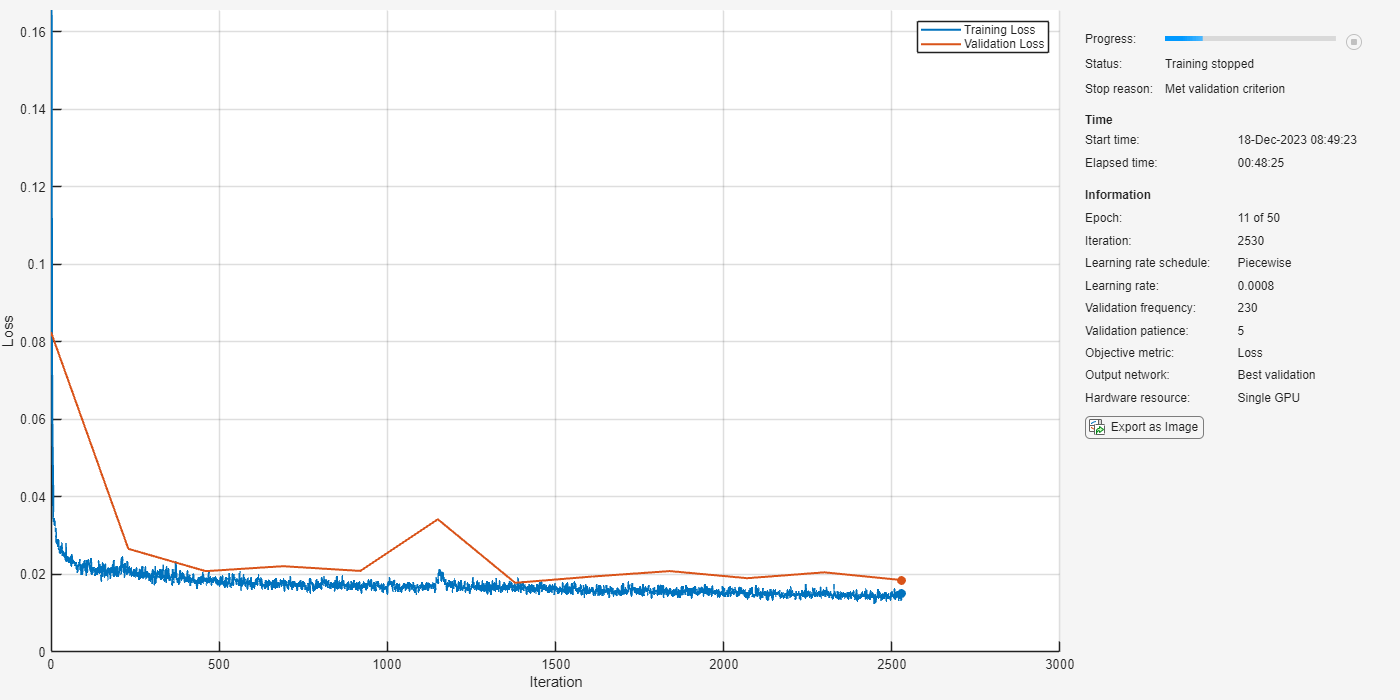### Evaluate Network Performance

#### Prediction and Reconstruction

Predict the log-magnitude spectra of the validation set.

`predictedSTFT4D = predict(dereverbNet,valReverb);`

Use the helperReconstructPredictedAudios function to reconstruct the predicted speech. This function performs actions outlined in the first section.

```params.WindowdowLength = 512; params.Window = hamming(params.WindowdowLength,"periodic"); params.OverlapLength = round(0.75*params.WindowdowLength); params.FFTLength = params.WindowdowLength; params.fs = 16000; dereverbedAudioAll = helperReconstructPredictedAudios(predictedSTFT4D,valMinMaxPairs,allValReverbPhase,allValReverbAudios,params);```

Visualize the log-magnitude STFTs of the clean, reverberant, and corresponding dereverberated speech signals.

```figure(Position=[100,100,1024,1200]) tiledlayout(3,1) nexttile imagesc(squeeze(allValCleanFeatures{1})) set(gca,Ydir="normal") subtitle("Clean") xlabel("Time") ylabel("Frequency") colorbar nexttile imagesc(squeeze(allValReverbFeatures{1})) set(gca,Ydir="normal") subtitle("Reverberated") xlabel("Time") ylabel("Frequency") colorbar nexttile imagesc(squeeze(predictedSTFT4D(:,:,:,1))) set(gca,Ydir="normal") subtitle("Predicted (Dereverberated)") xlabel("Time") ylabel("Frequency") clim([-1,1]) colorbar```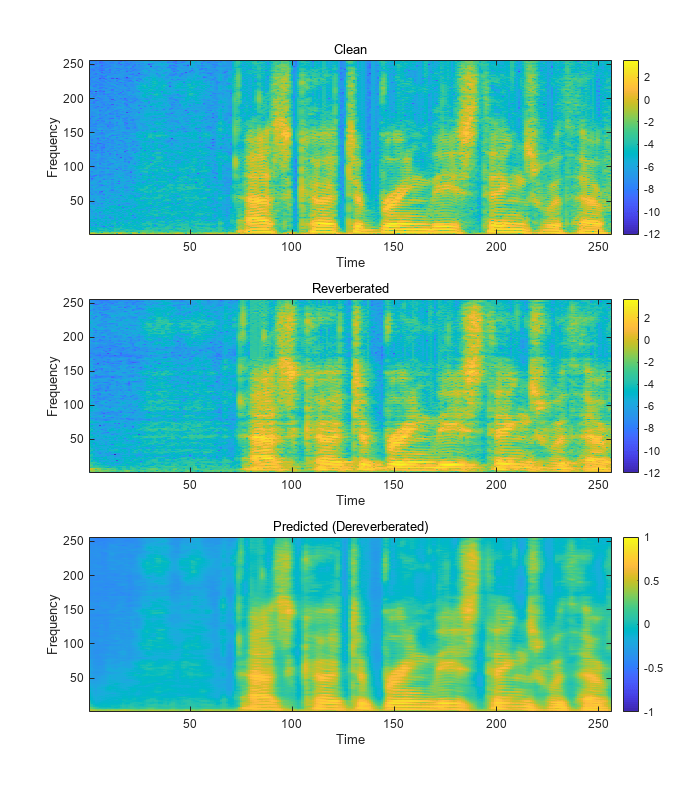#### Evaluation Metrics

You will use a subset of objective measures used in  to evaluate the performance of the network. These metrics are computed on the time-domain signals.

• Cepstrum distance (CD) - Provides an estimate of the log spectral distance between two spectra (predicted and clean). Smaller values indicate better quality.

• Log likelihood ratio (LLR) - Linear predictive coding (LPC) based objective measurement. Smaller values indicate better quality.

Compute these measurements for the reverberant speech and the dereverberated speech signals.

```[summaryMeasuresReconstructed,allMeasuresReconstructed] = calculateObjectiveMeasures(dereverbedAudioAll,allValCleanAudios,params.fs); [summaryMeasuresReverb,allMeasuresReverb] = calculateObjectiveMeasures(allValReverbAudios,allValCleanAudios,params.fs); disp(summaryMeasuresReconstructed)```
``` avgCdMean: 3.8310 avgCdMedian: 3.3536 avgLlrMean: 0.9103 avgLlrMedian: 0.8007 ```
`disp(summaryMeasuresReverb)`
``` avgCdMean: 4.2591 avgCdMedian: 3.6336 avgLlrMean: 0.9726 avgLlrMedian: 0.8714 ```

The histograms illustrate the distribution of mean CD, mean SRMR and mean LLR of the reverberant and dereverberated data.

```figure(Position=[50,50,1100,1300]) tiledlayout(2,1) nexttile histogram(allMeasuresReverb.cdMean,10) hold on histogram(allMeasuresReconstructed.cdMean,10) subtitle("Mean Cepstral Distance Distribution") ylabel("Count") xlabel("Mean CD") legend("Reverberant (Original)","Dereverberated (Predicted)") nexttile histogram(allMeasuresReverb.llrMean,10) hold on histogram(allMeasuresReconstructed.llrMean,10) subtitle("Mean Log Likelihood Ratio Distribution") ylabel("Count") xlabel("Mean LLR") legend("Reverberant (Original)","Dereverberated (Predicted)")```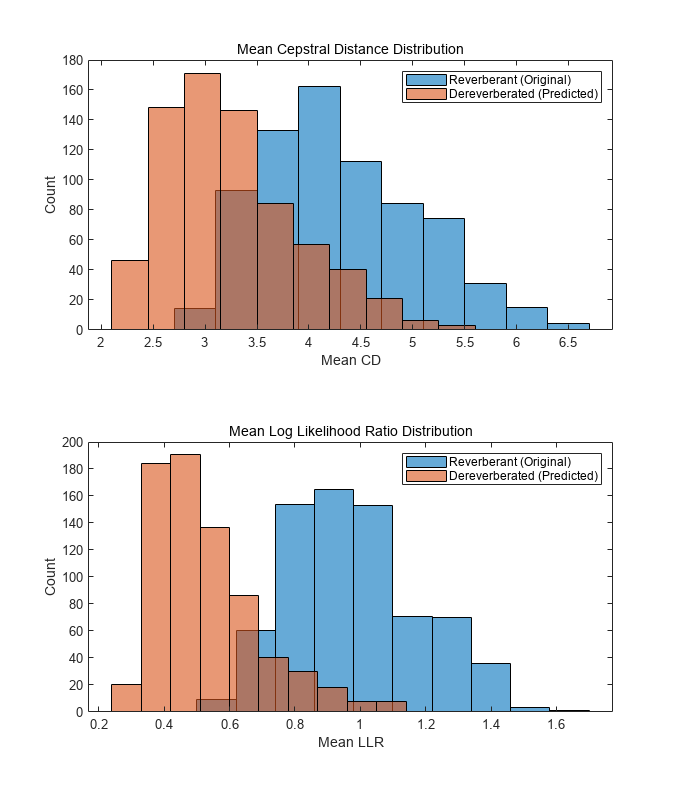### Supporting Functions

#### Apply Reverberation

```function yOut = applyReverb(y,preDelay,decayFactor,wetDryMix,fs) % This function generates reverberant speech data using the reverberator % object % % inputs: % y - clean speech sample % preDelay, decayFactor, wetDryMix - reverberation parameters % fs - sampling rate of y % % outputs: % yOut - corresponding reveberated speech sample revObj = reverberator(SampleRate=fs, ... DecayFactor=decayFactor, ... WetDryMix=wetDryMix, ... PreDelay=preDelay); yOut = revObj(y); yOut = yOut(1:length(y),1); end```

#### Extract Features Batch

```function [featuresClean,featuresReverb,phaseReverb,cleanAudios,reverbAudios] ... = helperFeatureExtract(cleanAudio,reverbAudio,isVal,params) % This function performs the preprocessing and features extraction task on % the audio files used for dereverberation model training and testing. % % inputs: % cleanAudio - the clean audio file (reference) % reverbAudio - corresponding reverberant speech file % isVal - Boolean flag indicating if it is the validation set % params - a structure containing feature extraction parameters % % outputs: % featuresClean - log-magnitude STFT features of clean audio % featuresReverb - log-magnitude STFT features of reverberant audio % phaseReverb - phase of STFT of reverberant audio % cleanAudios - 2.072s-segments of clean audio file used for feature extraction % reverbAudios - 2.072s-segments of corresponding reverberant audio assert(length(cleanAudio) == length(reverbAudio)); nSegments = floor((length(reverbAudio) - (params.samplesPerImage - params.shiftImage))/params.shiftImage); featuresClean = {}; featuresReverb = {}; phaseReverb = {}; cleanAudios = {}; reverbAudios = {}; nGood = 0; nonSilentRegions = detectSpeech(reverbAudio, params.fs); nonSilentRegionIdx = 1; totalRegions = size(nonSilentRegions, 1); for cid = 1:nSegments start = (cid - 1)*params.shiftImage + 1; en = start + params.samplesPerImage - 1; nonSilentSamples = 0; while nonSilentRegionIdx < totalRegions && nonSilentRegions(nonSilentRegionIdx, 2) < start nonSilentRegionIdx = nonSilentRegionIdx + 1; end nonSilentStart = nonSilentRegionIdx; while nonSilentStart <= totalRegions && nonSilentRegions(nonSilentStart, 1) <= en nonSilentDuration = min(en, nonSilentRegions(nonSilentStart,2)) - max(start,nonSilentRegions(nonSilentStart,1)) + 1; nonSilentSamples = nonSilentSamples + nonSilentDuration; nonSilentStart = nonSilentStart + 1; end nonSilentPerc = nonSilentSamples * 100 / (en - start + 1); silent = nonSilentPerc < 50; reverbAudioSegment = reverbAudio(start:en); if ~silent nGood = nGood + 1; cleanAudioSegment = cleanAudio(start:en); assert(length(cleanAudioSegment)==length(reverbAudioSegment),"Lengths do not match after chunking") % Clean Audio [featsUnit, ~] = featureExtract(cleanAudioSegment, params); featuresClean{nGood} = featsUnit; %#ok % Reverb Audio [featsUnit, phaseUnit] = featureExtract(reverbAudioSegment, params); featuresReverb{nGood} = featsUnit; %#ok if isVal phaseReverb{nGood} = phaseUnit; %#ok reverbAudios{nGood} = reverbAudioSegment;%#ok cleanAudios{nGood} = cleanAudioSegment;%#ok end end end end```

#### Extract Features

```function [features, phase, lastFBin] = featureExtract(audio, params) % Function to extract features for a speech file audio = single(audio); audioSTFT = stft(audio,Window=params.Window,OverlapLength=params.OverlapLength, ... FFTLength=params.FFTLength,FrequencyRange="onesided"); phase = single(angle(audioSTFT(1:end-1,:))); features = single(log(abs(audioSTFT(1:end-1,:)) + 10e-30)); lastFBin = audioSTFT(end,:); end```

#### Normalize and Reshape Features

```function [featNorm,minMaxPairs] = featureNormalizeAndReshape(feats) % function to normalize features - range [-1, 1] and reshape to 4 % dimensions % % inputs: % feats - 3-dimensional array of extracted features % % outputs: % featNorm - normalized and reshaped features % minMaxPairs - array of original min and max pairs used for normalization nSamples = length(feats); minMaxPairs = zeros(nSamples,2,"single"); featNorm = zeros([size(feats{1}),nSamples],"single"); parfor i = 1:nSamples feat = feats{i}; maxFeat = max(feat,[],"all"); minFeat = min(feat,[],"all"); featNorm(:,:,i) = 2.*(feat - minFeat)./(maxFeat - minFeat) - 1; minMaxPairs(i,:) = [minFeat,maxFeat]; end featNorm = reshape(featNorm,size(featNorm,1),size(featNorm,2),1,size(featNorm,3)); end```

#### Reconstruct Predicted Audio

```function dereverbedAudioAll = helperReconstructPredictedAudios(predictedSTFT4D,minMaxPairs,reverbPhase,reverbAudios,params) % This function will reconstruct the 2.072s long audios predicted by the % model using the predicted log-magnitude spectrogram and the phase of the % reverberant audio file % % inputs: % predictedSTFT4D - Predicted 4-dimensional STFT log-magnitude features % minMaxPairs - Original minimum/maximum value pairs used in normalization % reverbPhase - Array of phases of STFT of reverberant audio files % reverbAudios - 2.072s-segments of corresponding reverberant audios % params - Structure containing feature extraction parameters predictedSTFT = squeeze(predictedSTFT4D); denormalizedFeatures = zeros(size(predictedSTFT),"single"); for ii = 1:size(predictedSTFT,3) feat = predictedSTFT(:,:,ii); maxFeat = minMaxPairs(ii,2); minFeat = minMaxPairs(ii,1); denormalizedFeatures(:,:,ii) = (feat + 1).*(maxFeat-minFeat)./2 + minFeat; end predictedSTFT = exp(denormalizedFeatures); nCount = size(predictedSTFT,3); dereverbedAudioAll = cell(1,nCount); nSeg = params.NumSegments; win = params.Window; ovrlp = params.OverlapLength; FFTLength = params.FFTLength; parfor ii = 1:nCount % Append zeros to the highest frequency bin stftUnit = predictedSTFT(:,:,ii); stftUnit = cat(1,stftUnit, zeros(1,nSeg)); phase = reverbPhase{ii}; phase = cat(1,phase,zeros(1,nSeg)); oneSidedSTFT = stftUnit.*exp(1j*phase); dereverbedAudio = istft(oneSidedSTFT, ... Window=win,OverlapLength=ovrlp, ... FFTLength=FFTLength,ConjugateSymmetric=true,... FrequencyRange="onesided"); dereverbedAudioAll{ii} = dereverbedAudio./max(max(abs(dereverbedAudio)),max(abs(reverbAudios{ii}))); end end```

#### Calculate Objective Measures

```function [summaryMeasures,allMeasures] = calculateObjectiveMeasures(reconstructedAudios,cleanAudios,fs) % This function computes the objective measures on time-domain signals. % % inputs: % reconstructedAudios - An array of audio files to evaluate. % cleanAudios - An array of reference audio files % fs - Sampling rate of audio files % % outputs: % summaryMeasures - Global means of CD, LLR individual mean and median values % allMeasures - Individual mean and median values nAudios = length(reconstructedAudios); cdMean = zeros(nAudios,1); cdMedian = zeros(nAudios,1); llrMean = zeros(nAudios,1); llrMedian = zeros(nAudios,1); parfor k = 1 : nAudios y = reconstructedAudios{k}; x = cleanAudios{k}; y = y./max(abs(y)); x = x./max(abs(x)); [cdMean(k),cdMedian(k)] = cepstralDistance(x,y,fs); [llrMean(k),llrMedian(k)] = lpcLogLikelihoodRatio(y,x,fs); end summaryMeasures.avgCdMean = mean(cdMean); summaryMeasures.avgCdMedian = mean(cdMedian); summaryMeasures.avgLlrMean = mean(llrMean); summaryMeasures.avgLlrMedian = mean(llrMedian); allMeasures.cdMean = cdMean; allMeasures.llrMean = llrMean; end```

#### Cepstral Distance

```function [meanVal, medianVal] = cepstralDistance(x,y,fs) x = x/sqrt(sum(x.^2)); y = y/sqrt(sum(y.^2)); width = round(0.025*fs); shift = round(0.01*fs); nSamples = length(x); nFrames = floor((nSamples - width + shift)/shift); win = window(@hanning,width); winIndex = repmat((1:width)',1,nFrames) + repmat((0:nFrames - 1)*shift,width,1); xFrames = x(winIndex).*win; yFrames = y(winIndex).*win; xCeps = cepstralReal(xFrames,width); yCeps = cepstralReal(yFrames,width); dist = (xCeps - yCeps).^2; cepsD = 10/log(10)*sqrt(2*sum(dist(2:end,:),1) + dist(1,:)); cepsD = max(min(cepsD,10),0); meanVal = mean(cepsD); medianVal = median(cepsD); end```

#### Real Cepstrum

```function realC = cepstralReal(x,width) width2p = 2^nextpow2(width); powX = abs(fft(x,width2p)); lowCutoff = max(powX(:))*10^-5; powX = max(powX,lowCutoff); realC = real(ifft(log(powX))); order = 24; realC = realC(1:order + 1,:); realC = realC - mean(realC,2); end```

#### LPC Log-Likelihood Ratio

```function [meanLlr,medianLlr] = lpcLogLikelihoodRatio(x,y,fs) order = 12; width = round(0.025*fs); shift = round(0.01*fs); nSamples = length(x); nFrames = floor((nSamples - width + shift)/shift); win = window(@hanning,width); winIndex = repmat((1:width)',1,nFrames) + repmat((0:nFrames - 1)*shift,width,1); xFrames = x(winIndex).*win; yFrames = y(winIndex).*win; lpcX = realLpc(xFrames,width,order); [lpcY,realY] = realLpc(yFrames,width,order); llr = zeros(nFrames,1); for n = 1:nFrames R = toeplitz(realY(1:order+1,n)); num = lpcX(:,n)'*R*lpcX(:,n); den = lpcY(:,n)'*R*lpcY(:,n); llr(n) = log(num/den); end llr = sort(llr); llr = llr(1:ceil(nFrames*0.95)); llr = max(min(llr,2),0); meanLlr = mean(llr); medianLlr = median(llr); end```

#### Real Linear Prection Coefficients

```function [lpcCoeffs, realX] = realLpc(xFrames,width,order) width2p = 2^nextpow2(width); X = fft(xFrames,width2p); Rx = ifft(abs(X).^2); Rx = Rx./width; realX = real(Rx); lpcX = levinson(realX,order); lpcCoeffs = real(lpcX'); end```

### References

 Ernst, O., Chazan, S.E., Gannot, S., & Goldberger, J. (2018). Speech Dereverberation Using Fully Convolutional Networks. 2018 26th European Signal Processing Conference (EUSIPCO), 390-394.